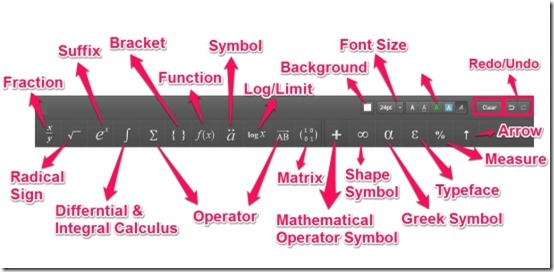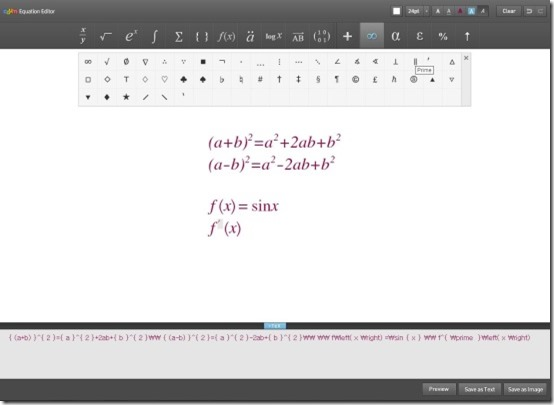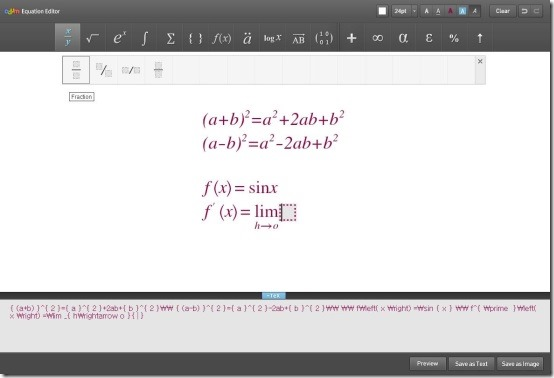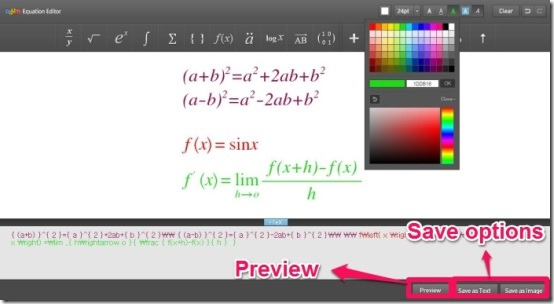Editor Ratings:
User Ratings:
[Total: 0 Average: 0]

Daum Equation Editor is a free equation editor offered by Chrome that enables you to quickly create and edit formula and save them as text or as an image. It offers you variety of symbols and notations that can be used for both simple and complex equations.

It enables you to add equations with special signs and also facilitates you to enter the numerical or variable values into the equation. It is an apt web app for teachers and students as it works in offline mode as well.

Daum Equation Editor facilitates you with an equation editing interface that lets you easily input equations either by typing on your keyboard and or by selecting symbols from its menu. It facilitates you to customize font size and provides you multiple color contrasts for text and background. It lets you save your equation as text or as an image. Let’s begin to create and edit formula with a text input editor.## How To Create and Edit Formula Using Daum Equation Editor?

Daum Equation Editor is a powerful and intelligent free equation editor, which can be grabbed by the link provided to you at the end of this article. You just need to add this app to your chrome browser. You can launch this app simply by clicking on Daum Equation Editor icon. As soon as you do that it facilitates you to create and edit formula with Tex input editor.

Daum Equation Editor enables you to write equations in two ways. Firstly, by typing on your keyboard and secondly, by selecting symbols from its menu. The Daum Equation Editor offers you a variety of symbols and notations that enables you to write basic and complex equations. It provides you options like fractions, suffix, log/limit, radical sign, differential and integral calculus, mathematical operator symbol and many more. This equation editor facilitates you with all the options as this visual editor provides you all that you need.You just have to begin to create any equation, as you are writing the equation in this equation editor it automatically converts the equation in the Tex format at the bottom. Like for example, if you wish to write a complex equation, you can simply do that using the Greek symbol for writing “f’(x)”.After that to complete the entire equation you can use Arrow to write “limit h->0” just by selecting the appropriate arrow from the menu bar of this equation editor.You can make use of fractions and other required signs and variables to complete the equation accordingly.Once you are done with creating the equations, you can simply select the equation and provide any color of your choice. Not only that, you can also change the size of the font and select the background according to your desire. You can now have the preview of the equations written in the visual editor and save them either as text or as an image.You may also read similar articles reviewed by us earlier: Lurch, LibreOffice and formulasheet.com

## Features Of Daum Equation Editor:

• Free equation editor offered by Google Chrome
• Enables you to quickly create and edit formula using Tex input editor
• Facilitates you to customize font size
• Offers you variety of symbols and notations
• Provides you multiple color contrasts for text and background
• Enables you to write both simple and complex equations
• It works in offline mode

## My Verdict For Daum Equation Editor:

Daum Equation Editor is a great web app for math and science teachers and students as it offers you variety of symbols and notations that can be used for both simple and complex equations. This equation editor app generates Tex code that you can use or reuse later. I liked the facility of color palette and saving the mathematical expressions as pictures, this will definitely help children to learn complex equations.